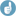## function sampleN.TOST of package PowerTOST [Power / Sample Size]

Hi Bryony,

❝ […]There are four-treatments (2xreference dosages & 2xtreatment dosages) & I plan to use the Williams' design…

❝ The coefficient of variation from previous studies is 20% & I am assuming the true test reference ratio to be between 0.95 and 1.05. I want to demonstrate bioequivalence (0.80-1.25) at 90% power at the 5% level.

I recommend the package PowerTOST for R (open source & free of costs).library(PowerTOST) sampleN.TOST(CV=0.2, theta0=0.95, theta1=0.8, theta2=1.25,              targetpower=0.9, alpha=0.05, design="4x4")

will give

+++++++++++ Equivalence test - TOST +++++++++++             Sample size estimation ----------------------------------------------- Study design:  4x4 crossover log-transformed data (multiplicative model) alpha = 0.05, target power = 0.9 BE margins = 0.8 ... 1.25 True ratio = 0.95,  CV = 0.2 Sample size (total)  n     power 24   0.907302

where n ist the total sample size (hence, six per sequence).

❝ […] if it was a standard AB/BA crossover, I estimate that I would require 12 individuals to complete each arm - is this correct? I am unsure how this calculation is extended to fit the 4x4 design - do I simply randomise 12 more individuals to each of the remaining two sequences?

Not quite so. In a 2×2 crossover we have n–2 degrees of freedom and in a 4×4 we have 3n–6. Sample size estimation is an iterative process (sample size is increased until at least the desired power is reached). In this process the degrees of freedom are important. Hence, multiplying the sample size for a 2×2 is not correct.

❝ Further, if I planned to have just 1 treatment dose changing the design as follows:

❝ ABC

❝ BCA

❝ CAB

❝ Is it reasonable to randomise 12 individuals per sequence?

No:

 sampleN.TOST(CV=0.2, theta0=0.95, theta1=0.8, theta2=1.25,              targetpower=0.9, alpha=0.05, design="3x3") +++++++++++ Equivalence test - TOST +++++++++++             Sample size estimation ----------------------------------------------- Study design:  3x3 crossover log-transformed data (multiplicative model) alpha = 0.05, target power = 0.9 BE margins = 0.8 ... 1.25 True ratio = 0.95,  CV = 0.2 Sample size (total)  n     power 24   0.904606

Therefore, 24 total or eight per sequence.

Be aware that simultaneous comparisons inflate the Type I Error (the patient’s risk). I guess that in your first design you will not compare the two test treatments but only T1 vs. R1, T1 vs. R2, T2 vs. R1, and T2 vs. R2. With these k=4 comparisons (each performed at the nominal α 0.05) the Familywise (Type I) Error Rate will be 1–(1–α)k≤18.55%. Bonferroni’s adjusted α will be α/k or 0.0125 which translates into a 100(1–2α/k) or 97.5% two-sided confidence interval whilst keeping the FWER with 1–(1–α/k)k≤4.91% below the nominal α 0.05. The lower α will substantially increase the sample size: 24 → 36

sampleN.TOST(CV=0.2, theta0=0.95, theta1=0.8, theta2=1.25,              targetpower=0.9, alpha=0.05/4, design="4x4") +++++++++++ Equivalence test - TOST +++++++++++             Sample size estimation ----------------------------------------------- Study design:  4x4 crossover log-transformed data (multiplicative model) alpha = 0.0125, target power = 0.9 BE margins = 0.8 ... 1.25 True ratio = 0.95,  CV = 0.2 Sample size (total)  n     power 36   0.918242

In your second design (T1 vs. R and T2 vs. R): 24 → 30

 sampleN.TOST(CV=0.2, theta0=0.95, theta1=0.8, theta2=1.25,              targetpower=0.9, alpha=0.05/2, design="3x3") +++++++++++ Equivalence test - TOST +++++++++++             Sample size estimation ----------------------------------------------- Study design:  3x3 crossover log-transformed data (multiplicative model) alpha = 0.025, target power = 0.9 BE margins = 0.8 ... 1.25 True ratio = 0.95,  CV = 0.2 Sample size (total)  n     power 30   0.909842

BTW, in your first design you opted for a Williams’ design

ABCD
BCDA
CDAB
DABC

and not a Latin Square

ABCD
BCDA
CDAB
DABC

In the second one for a Latin Square

ABC
BCA
CAB

and not a Williams’s design

ABC
BCA
CAB
ACB
BAC
CBA

Dif-tor heh smusma 🖖🏼 Довге життя Україна!Helmut SchützThe quality of responses received is directly proportional to the quality of the question asked. 🚮
Science QuotesIng. Helmut Schütz